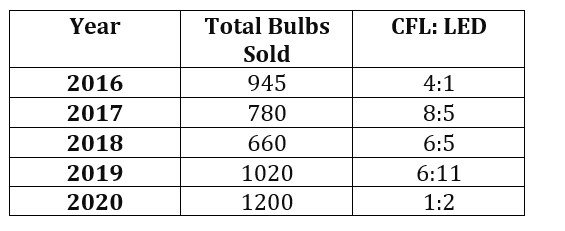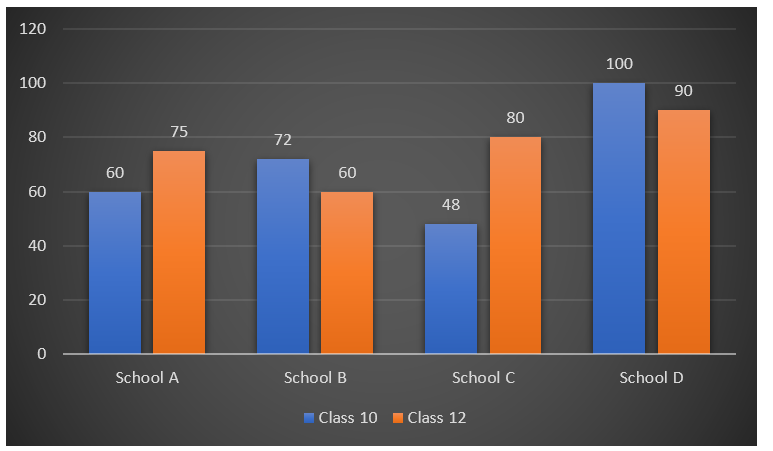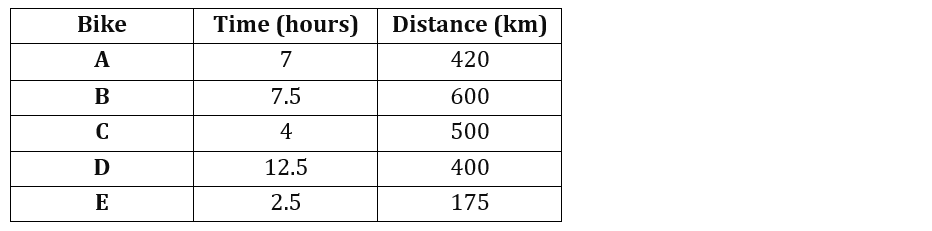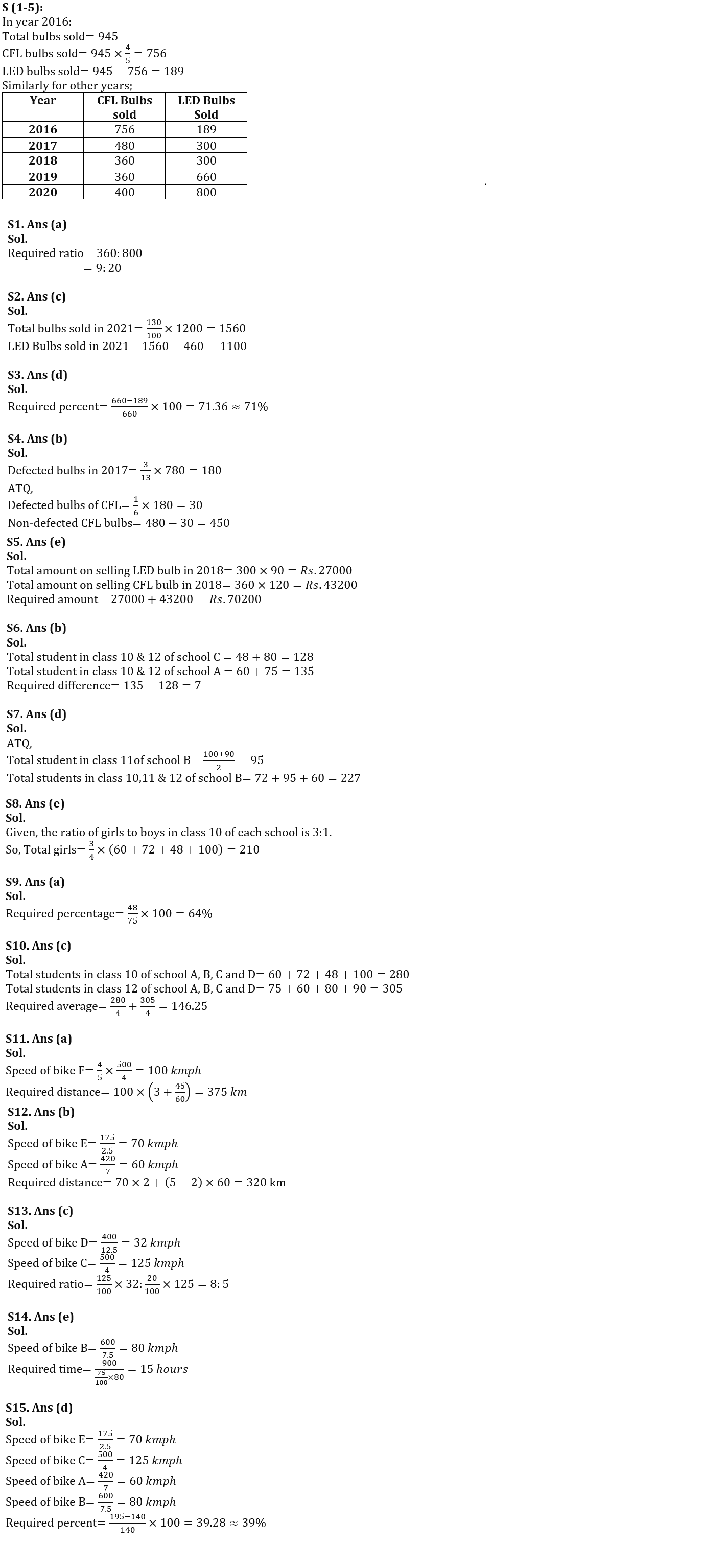Latest Banking jobs   »

# Quantitative Aptitude Quiz For Bank Foundation 2023- 26th January

Direction (1-5): Please understand the data carefully and answer the following questions.
Table below shows the total number of bulbs sold in five different years, i.e. 2016, 2017, 2018, 2019 and 2020. It also shows the ratio of CFL bulbs to LED bulbs sold in each year.
Note: Total bulbs sold=CFL bulbs+LED bulbsQ1. Find the respective ratio of number of CFL bulbs sold in 2019 to the number of LED bulbs sold in 2020?
(a)9: 20
(b)20: 9
(c)12: 5
(d)5: 12
(e)9: 5
Q2. If in 2021 the total bulbs sold was 30% more than the total bulbs sold in 2020 and the CFL bulbs sold in the same year are 460. Find the number of LED bulbs sold in 2021?
(a)720
(b)880
(c)1100
(d)940
(e)1260
Q3. Find the number of LED bulbs sold in 2016 is approximately what percent more/less than the total bulbs sold in 2018.
(a)47%
(b)53%
(c)65%
(d)71%
(e)82%
Q4. In the year 2017, the defected bulbs were 3/13 of total bulbs sold. If the ratio of CFL’s defected bulbs to LED’s defected bulbs is 1: 5, then find the number of non-defected CFL bulbs?
(a)400
(b)450
(c)470
(d)300
(e)360
Q5. If the cost of one CFL bulb and LED bulb sold in 2018 is Rs.120 and Rs.90 respectively, then find the total amount at which all bulbs were sold? (in Rs.)
(a)88400
(b)54100
(c)64600
(d)82300
(e)70200

Direction (6-10): Read the bar graph carefully and answer the following questions.
The bar graph below shows the total number of students in class in 10 and class 12 of four different school, i.e. A, B, C and D.Q6. Find the difference between the total student in Class 10 & 12 together of school C and class 10 & 12 together of school A?
(a)5
(b)7
(c)15
(d)13
(e)9
Q7. In school B the total students in class 11 are average of total students in class 10 and 12 together of school D. Find the total students in class 10,11 & 12 of school B?
(a)307
(b)327
(c)277
(d)227
(e)177
Q8. Find the total number of girls in all four school together of class 10, if the number of girls in class 10 of each school (A, B, C & D) is thrice the number of boys?
(a)155
(b)195
(c)180
(d)165
(e)210
Q9. Find the students in class 10 of school C is what percentage of the number of students in class 12 of school A?
(a)64%
(b)56%
(c)72%
(d)80%
(e)48%
Q10. What is the sum of average of total students who are in class 10 of all four schools and class 12 of all four schools together?
(a)167.25
(b)129.50
(c)146.25
(d)131.50
(e)110.50

Direction (11-15): Please understand the data carefully and answer the following questions. The table below shows the time taken and distance travelled by five different bikes, i.e. A, B, C, D and E.
Note: Distance travelled = Time taken × Speed of bikeQ11. If bike F’s speed is 4/5 of speed of bike C, then find the total distance travel by bike F in 3 hours and 45 minutes. (in km)
(a)375
(b)425
(c)350
(d)400
(e)325
Q12. If bike A runs at the speed of bike E for 2 hours and then runs at his usual speed, then find the distance travelled by him in 5 hours? (in km)
(a)220
(b)320
(c)420
(d)360
(e)260
Q13. Find the respective ratio of 125% of speed of bike D to the 20% of speed of bike C?
(a)5:7
(b)7:3
(c)8: 5
(d)5: 8
(e)3: 7
Q14. Find the time taken by B to complete a journey of 900 km if it runs at 25% less speed of itself only? (in hours)
(a)30
(b)20
(c)10
(d)25
(e)15
Q15. Find the combined speed of bike E and C together is approximately what percent more/less than the combined speed of bike A and B?
(a)29%
(b)47%
(c)33%
(d)39%
(e)43%

Solution## FAQs

### What is the selection process of the Bank Clerk?

The selection process of the Bank Clerk is Prelims & Mains.

#### Congratulations!Union Budget 2023-24: Free PDF